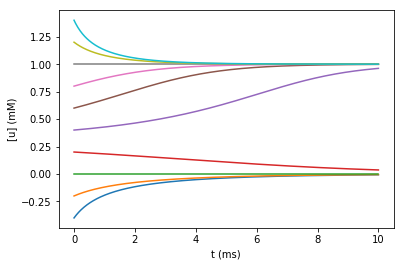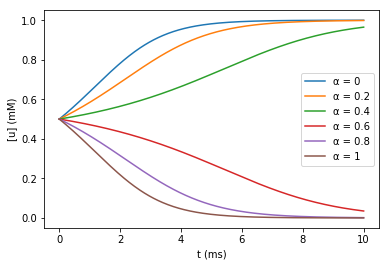# Reaction-Diffusion: varying initial concentrations and parameters

Often we will want to see how the choice of initial conditions affects the dynamics. We can do this by setting the initial attribute of an rxd.Species and rerunning.

For example, suppose at a single point we have the bistable dynamics introduced in the first part of this tutorial. That is, $u'=-u(1-u)(\alpha - u)$. (Here we use $u$ instead of a specific molecule name to indicate that we are not describing any particular molecule's kinetics, but rather modeling a class of phenomena.)

This time, we'll use an rxd.Parameter for $\alpha$ instead of a constant. This offers two advantages: (1) this allows $\alpha$ to vary spatially, and (2) this allows us to change the parameter values and rerun without changing the reaction/rate specification.

We start by defining the model and setting up the recordings:

In :
from neuron import h, rxd
from matplotlib import pyplot as plt

soma = h.Section(name='soma')
cyt = rxd.Region([soma], name='cyt', nrn_region='i')
u = rxd.Species(cyt, name='u')
α = rxd.Parameter(cyt, value=0.3)

rate = rxd.Rate(u, -u * (1 - u) * (α - u))

h.finitialize(-65)

t = h.Vector().record(h._ref_t)
y = h.Vector().record(soma(0.5)._ref_ui)

Out:



Now we define a function that sets the initial value for ca, runs the simulation, and plots the curves:

In :
def plot_it(ca_init):
u.initial = ca_init
h.finitialize(-65)
h.continuerun(10)
plt.plot(t, y)


And now let's run it a few times and plot it:

In :
for u0 in range(-4, 15, 2):
plot_it(u0 * 0.1)

plt.xlabel('t (ms)')
plt.ylabel('[u] (mM)')
plt.show()Here we see the stable fixed points at $0$ and $1$ and how all other trajectories (except for the one starting exactly at $\alpha$, not shown) tend towards one of those values.

Alternatively, we might want to know how the kinetics change if we start at a fixed value (say 0.5) and vary $\alpha$ instead. We can do this in the same way as above by setting the initial property of $\alpha$:

In :
def plot_α(α_init):
u.initial = 0.5
α.value = α_init
h.finitialize(-65)
h.continuerun(10)
plt.gca().plot(t, y, label='α = %g' % α_init)


Here we have added a label argument to the plot and explicitly specify the gca() axes to allow displaying a legend; see the matplotlib.pyplot.legend documentation for more.

Now we run it:

In :
for my_α in [0, 0.2, 0.4, 0.6, 0.8, 1]:
plot_α(my_α)

plt.gca().legend()
plt.xlabel('t (ms)')
plt.ylabel('[u] (mM)')
plt.show()